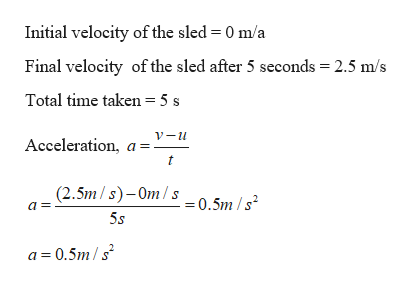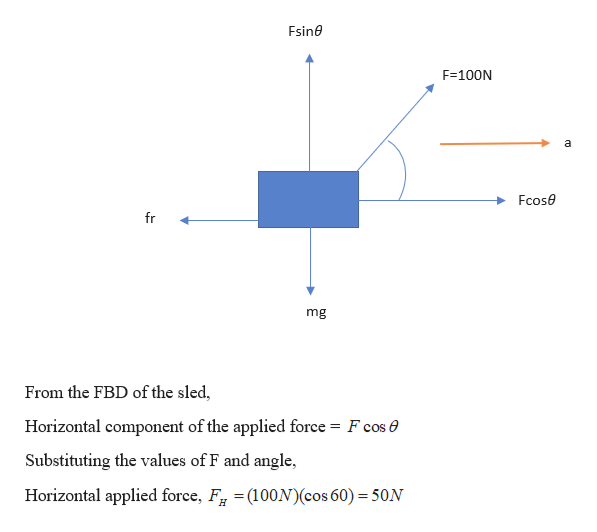# A 60kg sled is pulled by a string with a force of 100N and the string makes an angle of 60 degrees above the horizontal (up and to the right). The sled starts at rest and is moving at 2.5 m/s to the right after 5 seconds.1. Find the acceleration of the sled.1. Find the horizontal component of the pull force (Horizontal tension).2. Using the acceleration found in part 1 and the force in part 2, find the force of friction opposing the forward motion.

Question

60kg sled is pulled by a string with a force of 100N and the string makes an angle of 60 degrees above the horizontal (up and to the right). The sled starts at rest and is moving at 2.5 m/s to the right after 5 seconds.

1. Find the acceleration of the sled.

1. Find the horizontal component of the pull force (Horizontal tension).

2. Using the acceleration found in part 1 and the force in part 2, find the force of friction opposing the forward motion.

check_circle

Step 1

Given:

Mass of the sled = 60 kg

Force pulling the sled = 100 N at 60 degrees above horizontal

Velocity of sled after 5 seconds = 2.5 m/s

Step 2

(a) Calculating the acceleration of the sled:help_outlineImage TranscriptioncloseInitial velocity of the sled = 0 m/a Final velocity of the sled after 5 seconds 2.5 m/s Total time taken = 5 s Acceleration, a (2.5m/s)-Om/s = 0.5m /s2 5s a 0.5m/s fullscreen
Step 3

(b) Calculating the horizo...help_outlineImage TranscriptioncloseFsine F 100N a Fcose fr mg From the FBD of the sled, Horizontal component of the applied force = F cos 0 Substituting the values of F and angle, Horizontal applied force, F =(100N)(cos 60) 50N fullscreen

### Want to see the full answer?

See Solution

#### Want to see this answer and more?

Solutions are written by subject experts who are available 24/7. Questions are typically answered within 1 hour.*

See Solution
*Response times may vary by subject and question.
Tagged in

### Physics